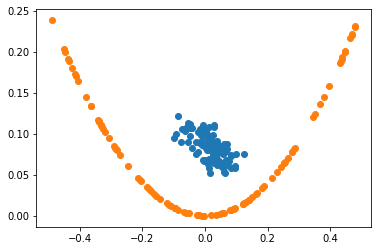# Is there anything obviously wrong with my very simple vanilla GAN?

Hello!

I want to train a GAN that is able to output pairs of the form (x, x²), simple as that.

First, In the following, we define the discriminator, the generator and some true samples of the form (x, x²) where x varies from -0.5 to 0.5.

``````import torch
import numpy as np
import matplotlib.pyplot as plt

class SampleGenerator:
def __init__(self, function, value_range):
self.function = function
self.range = value_range

def generate(self, n_samples):
x = (self.range - self.range)*np.random.rand(n_samples) + self.range
x = x.reshape(-1,1)
return (np.hstack([x, self.function(x)]), np.array(n_samples*).reshape(-1,1))

class Discriminator(torch.nn.Module):
def __init__(self):
super().__init__()
self.design = torch.nn.Sequential(
torch.nn.Linear(2, 10),
torch.nn.PReLU(),
torch.nn.Linear(10, 1),
torch.nn.Sigmoid()
)

def forward(self, x):
return self.design(x)

class Generator(torch.nn.Module):
def __init__(self):
super().__init__()
self.design = torch.nn.Sequential(
torch.nn.Linear(5, 20),
torch.nn.PReLU(),
torch.nn.Linear(20, 20),
torch.nn.PReLU(),
torch.nn.Linear(20, 2),
)

def forward(self, x):
return self.design(x)

s = SampleGenerator(lambda x: x**2, (-0.5, 0.5))
X_true, y_true = s.generate(100)

d = Discriminator()
doptimizer = torch.optim.Adam(d.parameters(), lr=0.0002, betas=(0.5, 0.999))

g = Generator()
goptimizer = torch.optim.Adam(g.parameters(), lr=0.0002, betas=(0.5, 0.999))

criterion = torch.nn.BCELoss()

X_true = torch.FloatTensor(X_true)
y_true = torch.FloatTensor(y_true)
y_fake = torch.FloatTensor(len(y_true)*).view(-1,1)

# Now the crucial training step.

for epoch in range(10000):
d.train()
g.train()

# Train discriminator
# ... with real examples
output = d(X_true)
dloss_real = criterion(output, y_true)
dloss_real.backward()
d_x = output.mean().item()

# ... with fake examples
seed = torch.randn(100, 5)
X_fake = g(seed)
output = d(X_fake)
dloss_fake = criterion(output, y_fake)
dloss_fake.backward()
dloss = dloss_real + dloss_fake
d_gz = output.mean().item()
doptimizer.step()

# Train Generator
output = d(g(seed))
gloss = criterion(output, y_true)
gloss.backward()
goptimizer.step()

if epoch%10==0:
print(d_x, d_gz)

# I let it run and run and run and in the end, the generated sample output looks quite bad.

seed = torch.rand(100,5)
plt.scatter(g(seed).detach().numpy()[:, 0], g(seed).detach().numpy()[:, 1])
plt.scatter(X_true[:, 0], X_true[:, 1])
``````Is there anything obviously wrong with what I’ve done? I also noticed, that the errors that I output are always around 0.5. I have understodd it like d_x should start at 1 and decrease towards 0.5, while d_gz should start at 0 and increase towards 0.5 in the training process. But here both start off at the goal. Why?

Thank you very much!

Sincerely
Garve